Courses

# Solutions of Electricity (Page No- 43) - Physics By Lakhmir Singh, Class 10 Class 10 Notes | EduRev

## Class 10 : Solutions of Electricity (Page No- 43) - Physics By Lakhmir Singh, Class 10 Class 10 Notes | EduRev

The document Solutions of Electricity (Page No- 43) - Physics By Lakhmir Singh, Class 10 Class 10 Notes | EduRev is a part of the Class 10 Course Class 10 Physics Solutions By Lakhmir Singh & Manjit Kaur.
All you need of Class 10 at this link: Class 10

Lakhmir Singh Physics Class 10 Solutions Page No:43

Question 43:
Show with the help of diagrams, how you would connect three resistors each of resistance 6 Ω, so that the combination has resistance of (i) 9 Ω (ii) 4 Ω.
Solution :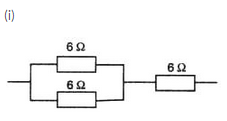Resultant resistance for parallel circuit=R

1/R = 1/6 + 1/6

1/R = 2/6 R = 3

Effective resistance = 6 + 3 = 9ohms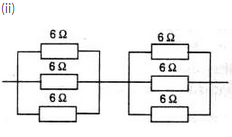Resultant resistance for each parallel circuit= R

1/R = 1/6+1/6+1/6

1/R = 3/6

R = 2

Therefore effective resistance = 2+2 = 4ohms.

Question 44:
Two resistances when connected in parallel give resultant value of 2 ohm; when connected in series the value becomes 9 ohm. Calculate the value of each resistance.
Solution :
Two resistances when connected in series, resultant value is 9ohms.

Two resistances when connected in parallel, resultant value is 2ohms.

Let the two resistances be R1 and R2.

If connected in series, then

9 = R1+ R2

R1 = 9-R2

If connected in parallel, then

1/2 = 1/R1+1/R2

From above equations we get that

1/2=(R1+R2)/R1R2

1/2 = 9/(9-R2) R2

9R2-R2= 18

R22-9R2+18 = 0

(R2-6) (R2-3)=0

R2 = 6,3

So if R2 = 6ohms, then R= 9-6 =3ohms.

If R2 = 3ohms, then R1= 9-3 = 6ohms.

Question 45:
A resistor of 8 ohms is connected in parallel with another resistor X. The resultant resistance of the combination is 4.8 ohms. What is the value of the resistor X ?
Solution :

Given:

A resistor of 8ohm is connected in parallel with a resistor of X.

And resultant is 4.8.

Then  X = ?

We know that for parallel case

1/R = 1/R1+1/X
1/4.8 = 1/8+1/x
1/4.8 - 1/8 = 1/x

After solving we get that

X = 12ohms

Question 46:
You are given three resistances of 1,2 and 3 ohms. Show by diagrams, how with the help of these resistances you can get:
(i) 6 Ω (ii) [latex s=2]\frac{6}{11}[/latex] Ω (iii) 1.5 Ω
Solution :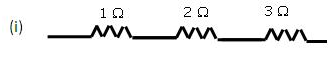Equivalent resistance = 10 + 20 + 30 = 60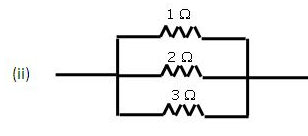Equivalent resistance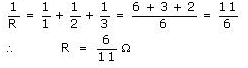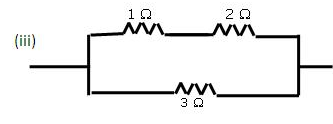Equivalent resistance of first line = 1Ω + 2Ω = 3Ω
Resistance of the second line = 3 0 Equivalent resistance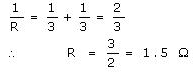Offer running on EduRev: Apply code STAYHOME200 to get INR 200 off on our premium plan EduRev Infinity!

94 docs

,

,

,

,

,

,

,

,

,

,

,

,

,

,

,

,

,

,

,

,

,

,

,

,

;